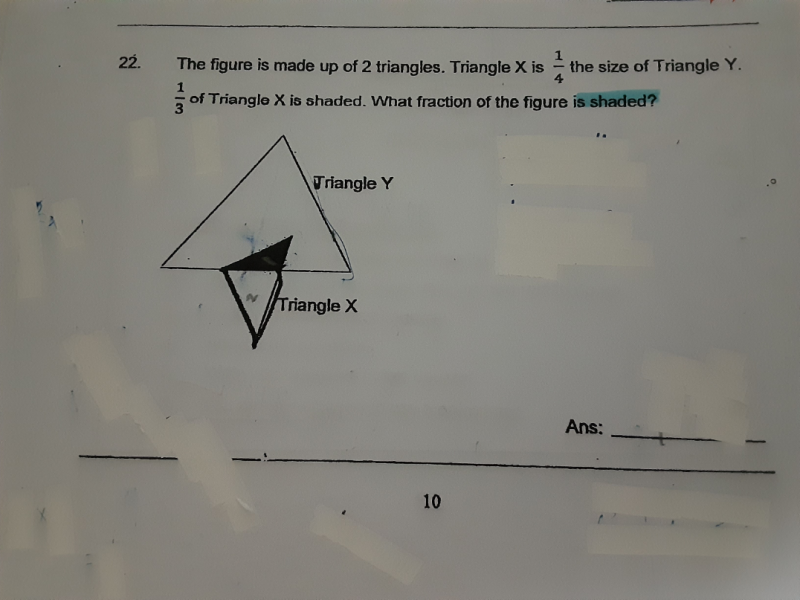# QuestionDear parents

Pls help me on this question thank you

Since the shaded portion of triangle X is 1/3 that of the whole triangle X, this means we can take triangle X as 3 units.

We also know that triangle X is 1/4 that of triangle Y. Therefore, triangle Y will be (3 × 4 = 12) units.

The whole figure is then 12 + 3 – 1 = 14 units. We minus 1 unit because the shaded portion is an overlap between the two triangles and should not be counted twice.

So ratio of shaded portion  to whole figure is 1 : 14 or 1/14 as a fraction.

0 Replies 1 Like

For Triangle X.
1 : 2 : 3

Triangle X : Triangle Y
1 : 4
3 : 12

For Triangle Y,